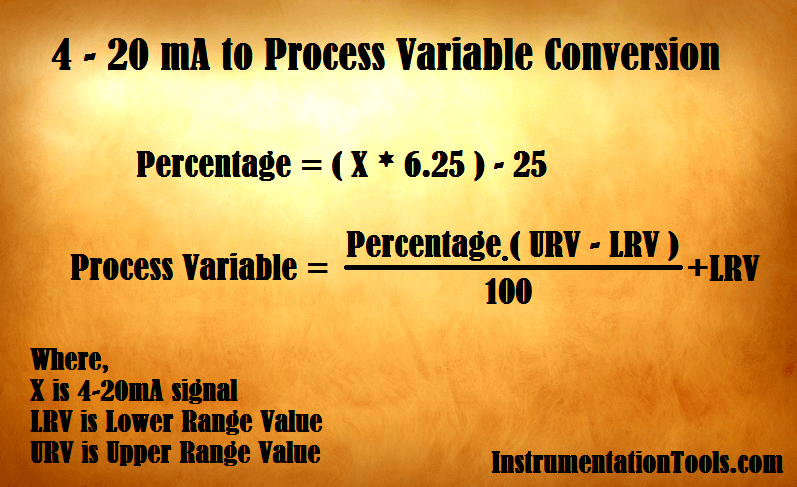# Formula to Calculate Process Variable from 4-20mA

How to Calculate Process Variable from 4-20mA current loop output of a field transmitter. Check the formula to find out Process variable (PV).

## Calculate Process Variable from 4-20mA##### Formula :

First Calculate equivalent Percentage from 4-20mA signal

#### Percentage = ( X * 6.25 ) – 25

Then calculate equivalent Process variable from percentage

#### Process Variable =  [ ( Percentage * ( URV – LRV ) /100 ) ] + LRV

Where,

X is 4-20mA signal

LRV is Lower Range Value

URV is Upper Range Value

Example :

### A Temperature transmitter with a range of 0 to 250 deg C and its output is 15.5 mA. Calculate equivalent temperature value.

Lower Range Value, LRV = 0

Upper Range Value, URV = 250

Output Current in range of 4-20mA, X = 15.5 mA

Percentage = ( X * 6.25 ) – 25 = (15.5 * 6.25) – 25 = 71.875 %

Process Variable = [ { Percentage * ( URV – LRV ) } / 100 ] + LRV

Process Variable = [ ( 71.875 * 250 ) / 100 ] + 0 = 179.6875

Also you can use online Instrumentation Calculator for Automatic calculations. Click Here.

#### Articles You May Like :

Control System Setpoints & Alarms

4-20mA Transmitter Operation

Transmitter Turndown Ratio

Calculate 4-20 mA from PV

Linear mA to Square root mA

Be the first to get exclusive content straight to your email.
We promise not to spam you. You can unsubscribe at any time.

### 4 thoughts on “Formula to Calculate Process Variable from 4-20mA”

1.Can you help please. This week I installed a vibration sensor with a 4-20mA output. At the data logger I burdened the loop with a 100 ohm precision resistor. Unfortunately the logger was setup for a 500 ohm resistor. The range should have been 0-20 mm/sec for .4 to 2v. Instead, because of the wrong resistor, the range was -5 to 0. Could you tell me please how to re-scale -5-0 to 0-20 ?

2.thank u sir for detail information

3.I don’t really know what 6.25 and 25 figures in the first formula.
But the easiest way is:
1) to subtract 4 from 15.5.
4-15.5= 11.5
2) Then divide 11.5 by 16 “Span” and multiply by 100.
(11.5\16)*100= 71.875%

Simple to remember and to do.
??

4.